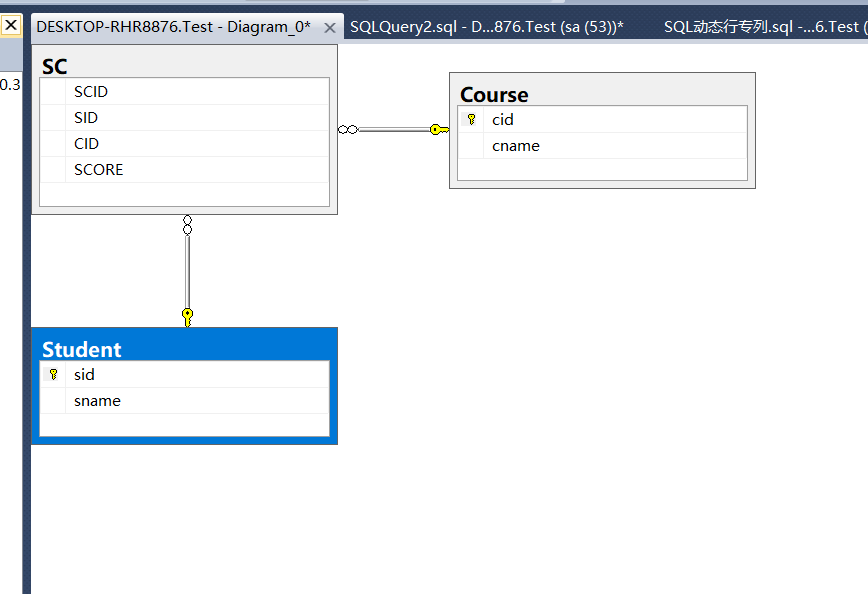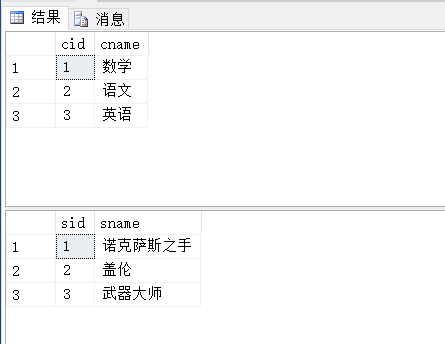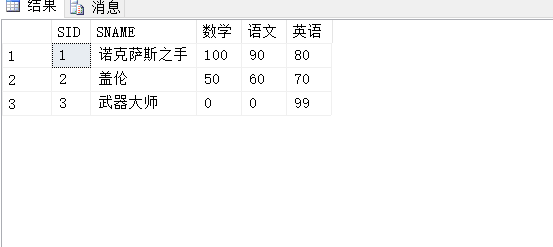# SQL行转列应用的动态实现方式

lancelottsang

0收藏

SQL行转列的需求，在项目中还是经常可见的，尤其报表类的应用，更是非常广泛!上期我们讲了SQL行转列的静态实现方式，本期搞一下行转列的动态实现方案，解决方案并不唯一，这里采用存储过程的实现方式!``````SELECT S.SID,S.sname,
MAX(case c.cname when '数学' then sc.score else 0 end) as 数学,
MAX(case c.cname when '语文' then sc.score else 0 end) as 语文,
MAX(case c.cname when '英语' then sc.score else 0 end) as 英语
FROM Student as S
LEFT JOIN SC AS SC ON S.sid = SC.SID
LEFT JOIN Course AS C ON C.cid = SC.CID
GROUP BY S.sid,S.sname````````````DECLARE @SQL VARCHAR(MAX)
SELECT @SQL = ' SELECT S.SID,S.SNAME '
SELECT @SQL = @SQL + ' , ISNULL(MAX(CASE c.cname WHEN '''+cname+''' THEN sc.score END ),0) AS '''+c.cname+''' '
FROM Course  AS C
print @sql
SELECT @SQL = @SQL + ' FROM Student as S
LEFT JOIN SC AS SC ON S.sid = SC.SID
LEFT JOIN Course AS C ON C.cid = SC.CID
GROUP BY S.sid,S.sname'
print @sql
EXEC (@SQL)``````

``````CREATE PROC StudentScore_Proc
AS
BEGIN
DECLARE @SQL NVARCHAR(MAX)
SELECT @SQL = N' SELECT S.SID,S.SNAME '
SELECT @SQL = @SQL + N' , ISNULL(MAX(CASE c.cname WHEN '''+cname+''' THEN sc.score END ),0) AS '''+c.cname+''' '
FROM Course  AS C
SELECT @SQL = @SQL + N' FROM Student as S
LEFT JOIN SC AS SC ON S.sid = SC.SID
LEFT JOIN Course AS C ON C.cid = SC.CID
GROUP BY S.sid,S.sname'
print @sql
EXECUTE sp_executesql
@STMT = @SQL
END
``````

``````INSERT INTO Course SELECT 4,'物理'
INSERT INTO Course SELECT 5,'化学'
INSERT INTO SC SELECT 1,4,99
INSERT INTO SC SELECT 1,5,88
INSERT INTO SC SELECT 2,5,77
EXEC dbo.StudentScore_Proc``````帖子
视频
声望
粉丝
社区精华内容